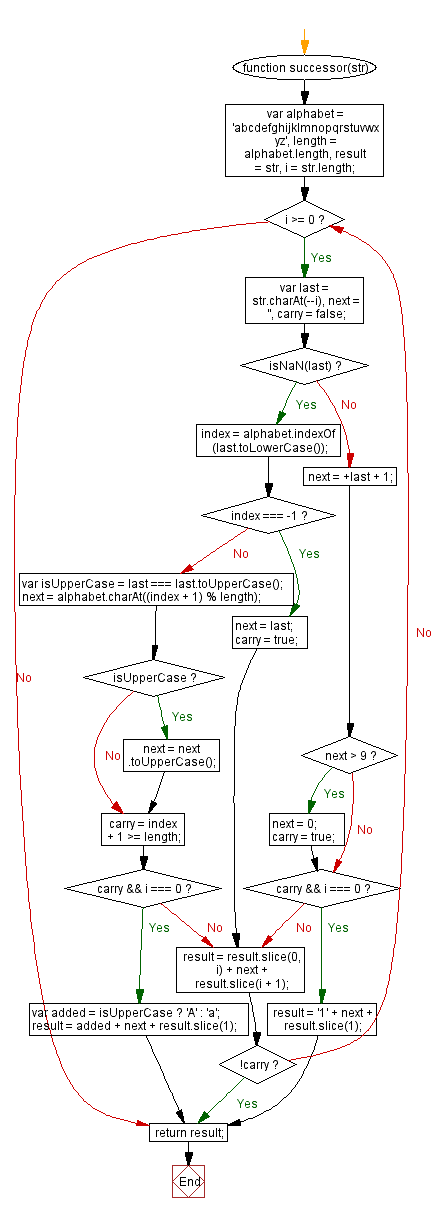# JavaScript: Get the successor of a string

## JavaScript String: Exercise-48 with Solution

Write a JavaScript function to get the successor of a string.

Note: The successor is calculated by incrementing characters starting from the rightmost alphanumeric (or the rightmost character if there are no alphanumerics) in the string. Incrementing a digit always results in another digit, and incrementing a letter results in another letter of the same case. If the increment generates a carry, the character to the left of it is incremented. This process repeats until there is no carry, adding an additional character if necessary.

Example:
string.successor("abcd") == "abce"
string.successor("THX1138") == "THX1139"
string.successor("< >") == "< >"
string.successor("1999zzz") == "2000aaa"
string.successor("ZZZ9999") == "AAAA0000"

Test Data:
console.log(successor('abcd'));
console.log(successor('3456'));
"abce"
"3457"

Sample Solution:-

HTML Code:

``````<!DOCTYPE html>
<html>
<meta charset="utf-8">
<title>JavaScript function to get the successor of a string</title>
<body>

</body>
</html>
```
```

JavaScript Code:

``````function successor(str) {
var alphabet = 'abcdefghijklmnopqrstuvwxyz',
length = alphabet.length,
result = str,
i = str.length;

while(i >= 0) {
var last = str.charAt(--i),
next = '',
carry = false;

if (isNaN(last)) {
index = alphabet.indexOf(last.toLowerCase());

if (index === -1) {
next = last;
carry = true;
}
else {
var isUpperCase = last === last.toUpperCase();
next = alphabet.charAt((index + 1) % length);
if (isUpperCase) {
next = next.toUpperCase();
}

carry = index + 1 >= length;
if (carry && i === 0) {
var added = isUpperCase ? 'A' : 'a';
result = added + next + result.slice(1);
break;
}
}
}
else {
next = +last + 1;
if(next > 9) {
next = 0;
carry = true;
}

if (carry && i === 0) {
result = '1' + next + result.slice(1);
break;
}
}

result = result.slice(0, i) + next + result.slice(i + 1);
if (!carry) {
break;
}
}
return result;
}

console.log(successor('abcd'));
console.log(successor('3456'));
```
```

Sample Output:

```abce
3457
```

Flowchart:Live Demo:

See the Pen JavaScript Get the successor of a string-string-ex-48 by w3resource (@w3resource) on CodePen.

Improve this sample solution and post your code through Disqus

What is the difficulty level of this exercise?

Test your Programming skills with w3resource's quiz.

﻿

## JavaScript: Tips of the Day

Tagged template literals

```function getPersonInfo(one, two, three) {
console.log(one);
console.log(two);
console.log(three);
}

const person = 'Lydia';
const age = 21;

getPersonInfo`\${person} is \${age} years old`;
```

If you use tagged template literals, the value of the first argument is always an array of the string values. The remaining arguments get the values of the passed expressions!

Ref: https://bit.ly/323Y0P6